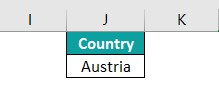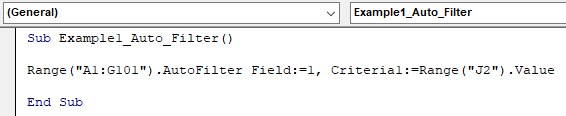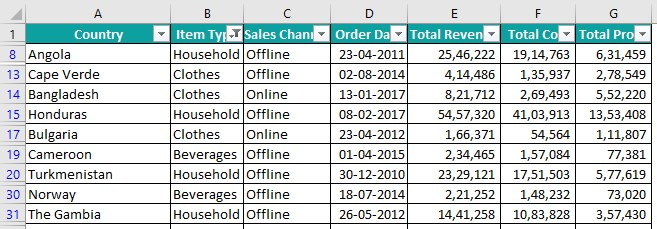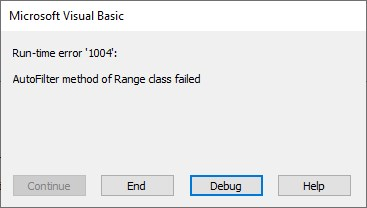# VBA AutoFilterArticle byJeevan A Y## Excel VBA AutoFilter

VBA AutoFilter is a feature that helps us to filter data based on various criteria and conditions. If we have a data set which has to be filtered based on various criteria, we can use the Filter option in the Data ribbon.

Similarly, we have an auto filter in VBA to perform various kinds of filtering on the data. In this article, we will take you through the entire process involved in the VBA auto filter.

###### Key Takeaways
• VBA Auto Filter works similarly to the Filter option in the worksheet.
• It filters the data from the given column number.
• We can filter more than one value from a single column by using the operator argument.
• Also, we can filter both text values as well as numerical values.
• We can check the status of the auto filter by using AutoFilterMode.
• VBA Auto Filter will throw the “Auto Filter method of Range class failed” error if the given column number is not in the range of the data.

### Syntax Of AutoFilter Function

The following is the syntax of the Auto Filter function.

Range(“Data Range”).AutoFilter( [Field] , [Criteria1] , [Operator] , [Criteria2] , [VisibleDropDown])

• Range(“Data Range”): First, we need to provide the data range to apply the filter using the RANGE object.

[Field]: [Optional Argument] In this argument, we need to provide the column number on which we are going to apply Auto Filter in Excel VBA. The column number should be counted from the left side of the data range.

[Criteria1]: [Optional Argument] In the given column number, we will provide the value we are going to filter in double quotes.

[Operator]: [Optional Argument] If we are going to use the [Criteria2] then we need to use this operator argument. We can combine criteria 1 and criteria 2 by using the operator symbols. The operators available for use in the operator argument are:

xlAnd, xlOr, xlBottom10Items, xlTop10Items, xlBottom10Percent, xlTop10Percent, xlFilterCellColor, xlFilterDynamic, xlFilterFontColor, xlFilterIcon, xlFilterValues

[Criteria2]: [Optional Argument] This is the second criteria based on which we filter the data along with the criteria1.

[VisibleDropDown]: [Optional Argument] Using this argument of the auto filter function, we can specify if the filter drop-down option should be visible or not on the filtering column. We can specify either TRUE or FALSE.

Since all the arguments are optional, if we do not specify arguments, then, it will just apply the filter icons (if filter icons are already there, then it will remove those icons).

If the filter is already applied on some columns, then it will remove all the filters from the given range and shows the full data.

### How To Apply Filter Using VBA AutoFilter?

Let’s look at some of the examples of applying VBA Auto Filter.

#### Example #1 – Filter Out Data By Using AutoFilter

For example, look at the following data that we will be using in all the examples of the VBA auto filter.

The data range is from A1 to G101, and we have 7 columns in the data table.

Assume we need to filter the data in the Sales Channel, with only the Online sales channel.

Step 1 – In the sub procedure, enter the RANGE object and provide the data range as A1:G101 in double quotes.

Step 2 – Once the range is provided, enter the dot and choose the Auto Filter option from the IntelliSense list.

Step 3 – After selecting the VBA Auto Filter option, we can see the following arguments of the auto filter function.

Step 4 – The first argument of the VBA auto filter function is Field i.e., on which column do we need to apply the filter?

Since we are applying the filter on the Sales Channel column, the column number will be 3 because, in the data range A1:G101, the sales channel column is the 3rd positioned column.

Step 5 – Next, we need to provide what value we are going to filter from the given column. As stated earlier, we are going to filter only the Online sales channel. So, for the Criteria1 argument, give the criteria as Online in double quotes.

Let’s run the code, and we will see filtered data as follows:

As we can see, it has applied the filter on the sales channel column, and we can see only the Online sales channel in the data range.

#### Example #2 – Operator In AutoFilter

Now we know how to apply the Auto Filter in VBA. Let’s explore more on the other arguments for the VBA Auto Filter.

For example, assume we need to filter the data based on Item Type now.

Step 1 – Assume we need to filter item types Household and Vegetables. We cannot provide these two filtering values in criteria 1 itself. Hence, we need to use the operator symbol to filter more than one item.

The field number will be 2 because the item type is the 2nd column in the data range given.

Step 2 – In the criteria1 argument provide the filtering value as Household.

Step 3 – Since we are going to filter one more value of the same column, we will use the operator argument input.

Enter the argument name Operator followed by colon (:) and equal sign (=) to the operator constants available in VBA.

We have various operator constants with the operator argument, and we can choose any one of them based on the requirement.

Step 4 – Since we are filtering two values, we will choose the xlOr operator symbol.

Step 5 – Next, for the Criteria2 argument, we can provide the input as Vegetables.

Step 6 – Let’s run the code, and it should filter the given values from the column Item Type.

It has filtered only values Household and Vegetables from the column Item Type.

#### Example #3 – Filter Numbers Using AutoFilter

Not only text values, but we can also filter numbers using VBA Auto Filter function in VBA. For example, let’s work with the same data from the previous 2 examples.

Step 1 – Assume we need to filter the data based on the profit column where the profit value is <10000. In the field, the argument provides the column as 7 because Profit is the 7th column number in the data range.

Step 2 – Next, for Criteria 1, give the condition as <10000.

Sub Example2_Auto_Filter()

``Range("A1:G101").AutoFilter Field:=7, Criteria1:="<10000"``

End Sub

This will filter the data based on the profit value where the values are less than 10000.

Step 3 – Similarly, to filter the values between 10000 and 30000, we can write the code as shown in the following image.

This will filter the profit values between 10000 and 30000.

#### Example #4 – Filter From Different Columns Using AutoFilter

We can filter values based on multiple columns as well. For example, assume we need to filter Online sales for items Fruits and Vegetables. Then, we can write the code in the following way.

Dim Rng As Range

First, we defined the variable and assigned the range object as the data type.

Set Rng = Range(“A1:G101”)

Since RANGE is an object data type, we use the VBA SET statement and assigned the range of cells for the variable Rng.

With Rng

.AutoFilter Field:=3, Criteria1:=”Online”

.AutoFilter Field:=2, Criteria1:=”Fruits”, Operator:=xlOr, Criteria2:=”Vegetables”

End With

Since we need to apply filters for more than one column, we use the WITH statement. In the first VBA Auto Filter, we have assigned the criteria for the 3rd (Sales Channel) column as Online. Next, for the second Auto Filter, we have assigned the filter for the 2nd column (Item Type) as either Fruits OR Vegetables.

This will apply the filter for Sales Channel and Item Type columns and filter the given criteria values.

### Turn Excel AutoFilter ON/OFF Using VBA

In the VBA Auto Filter function, all the arguments are optional. We must wonder what happens if none of the argument’s inputs are provided.

For example, look at the following code.

Sub Auto_Filter()

``Range("A1:G101").AutoFilter``

End Sub

We have not given any of the argument input for the VBA Auto Filter. And no filters have been applied.

For our reference, the following image shows how the data appeared before we run the code.

Let’s run the code and see what happens.

It has just applied filter icons on all the columns of the given data range.

Now, we will apply some filters for the columns.

Now let’s run the code and see what happens.

When we ran the code, it removed all the filters, including the filter icons on all the columns. So, we can use the VBA Auto Filter function without any arguments to turn on or off filters.

### Check If AutoFilter Is Already Applied

When we need to toggle between the VBA Auto Filter on and off, it is important to check if the Auto Filter is already applied. This is done using the Auto Filter mode option.

The following code will check if the Auto Filter is running in the active sheet.

Sub Check_Auto_Filter()

``````If ActiveSheet.AutoFilterMode = True Then
MsgBox "Auto Filter is already running"
Else
MsgBox "No auto fitlers are running"
End If``````

End Sub

The above code will return the following message box saying Auto Filter is already running if the Auto Filter is already turned on.

If no VBA Auto Filter is running, then it will return the following message box saying, No auto filters are running.

### Important Things To Note

• In VBA Auto filter, all the arguments are optional. If we do not enter any of the arguments, then it will turn on the auto filter in the given range if it is not running, or it will turn the Auto filter off, if it is already running.
• When we must filter the data based on two or more columns, then, we must use the WITH statement.
• VBA Auto Filter is available only with the range of cells using the Range object.
• It filters the data only in the given range of cells.

1. How do you Auto filter rows based on cell value in Excel VBA?

To filter data based on cell value, we need to first set up a criteria cell. For example, if we want to filter the data for the country Austria, then set up the criteria cell as shown in the following image.Then for the criteria, give the cell address as J2.Now, this will filter the data based on the J2 cell value.

2. Why is Auto Filter in Excel VBA Not Working?

Auto Filter usually throws an error if the column number provided is not within the data range. For example, in the following data, we have 6 columns.If we provide the field argument as 7, then, we will get the following error.So, the column numbers that we provide should be within the column count of the given data range.

3. How to clear all Auto Filter in Excel VBA?

To clear all auto filters, first, we need to check if the Auto Filter is on or not. The following code will check if the Auto Filter is on or not in the worksheet. If it is on, then it will remove the filter from the worksheet.

If ActiveSheet.AutoFilterMode Then
ActiveSheet.AutoFilterMode = False
End If

4. How to auto filter in VBA with criteria as a range of cells?

To Auto Filter with criteria with a range of cells, first, we need to set up criteria values in cells. Then, we need to use the array function to assign all the criteria values to the array variable and use the array variable name in the criteria argument of the array in the criteria argument of the Auto Filter function.